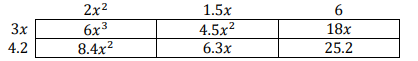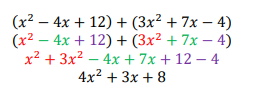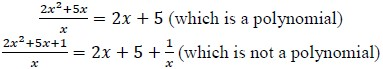# MA.912.AR.1.3Export Print
Add, subtract and multiply polynomial expressions with rational number coefficients.

### Clarifications

Clarification 1: Instruction includes an understanding that when any of these operations are performed with polynomials the result is also a polynomial.

Clarification 2: Within the Algebra 1 course, polynomial expressions are limited to 3 or fewer terms.

General Information
Subject Area: Mathematics (B.E.S.T.)
Strand: Algebraic Reasoning
Status: State Board Approved

## Benchmark Instructional Guide

• Polynomial

### Vertical Alignment

Previous Benchmarks

Next Benchmarks

### Purpose and Instructional Strategies

In middles grades, students added, subtracted and multiplied linear expressions. In Algebra I, students perform operations on polynomials limited to 3 or fewer terms. In later courses, students will perform operations on all polynomials.
• Instruction includes making the connection to dividing a polynomial by a monomial and the understanding that division does not have closure.
• Reinforce like terms during instruction (using different colors can be a strategy to help identify them as unique from one another).
• Instruction includes the use of manipulatives, like algebra tiles, and various strategies, like the area model, properties of exponents and the distributive property.
• Area model
• The expression (2$x$2 + 1.5$x$ + 6)(3$x$ + 4.2) is equivalent to 6$x$3 + 12.9$x$2  +  24.3$x$ + 25.2 and can be modeled below.• Instruction should not rely upon the use of tricks or acronyms, like FOIL.
• Although within the Algebra I course, polynomial expressions are limited to 3 or fewer terms, this restriction only refers to the expressions given to the student, not the expression after the operation applied.

### Common Misconceptions or Errors

• Students may not understand the meaning of closure or the operations it applies to with polynomials.
• Students may not understand like terms or the properties of exponents.

### Strategies to Support Tiered Instruction

• Instruction includes the use of shapes or colors to demonstrate like terms. Teacher must ensure that students understand that the sign in front of the terms are key when combining like terms.
• For example, different colors can be used when adding the polynomials shown below.• Teacher provides examples showing that polynomials are closed under the operations of addition, subtraction and multiplication, but not under division.
• For example, when subtracting or multiplying polynomials (as shown below), the result is always a polynomial.
7$x$ + 5 − (3$x$ + 8) = 4$x$ − 3
(7$x$ + 5)(3$x$ + 8) = 21$x$² + 71$x$ + 40
• For example, when dividing polynomials (as shown below), the result may or may not be a polynomial.• Part A. Determine the sum of 3$x$2 − 2$x$ +5  and $\frac{\text{1}}{\text{6}}$$x$2 + 7$x$$\frac{\text{8}}{\text{7}}$ . Explain the method used in determining the sum.
• Part B. Discuss whether the addition of polynomials will always result in another polynomial. Why or why not?
• Part C. Determine the difference of 3$x$3 −  2$x$2  +  5  and $x$2 – 0.25$x$ +  1.24. Explain the method used in determining the difference.
• Part D. Discuss whether the subtraction of polynomials will always result in another polynomial. Why or why not?
• Part E. Determine the product of 2$x$ + 5 and $\frac{\text{2}}{\text{9}}$$x$2 $\frac{\text{11}}{\text{2}}$$x$ + 1. Explain the method used in determining the product.
• Part F. Discuss whether the multiplication of polynomials will always result in another polynomial. Why or why not?
• Part G. Determine the quotient of 9$x$2 – 3$x$ + 12  and 3$x$. Explain the method used in determining the quotient.
• Part H. Discuss whether the division of polynomials will always result in another polynomial. Why or why not?

### Instructional Items

Instructional Item 1
• Determine the sum of the expression ($\frac{\text{3}}{\text{4}}$$x$3 − $\frac{\text{2}}{\text{3}}$) + (−$\frac{\text{1}}{\text{2}}$ $x$2 + $x$ + $\frac{\text{5}}{\text{6}}$).

Instructional Item 2

• Determine the value of the $x$² term when the expression ($x$2 + $\frac{\text{3}}{\text{4}}$$x$$\frac{\text{1}}{\text{2}}$) is multiplied by ($x$$\frac{\text{2}}{\text{3}}$).

Instructional Item 3
• Determine the difference of the expression (−0.4$x$ + 0.5$x$2 +2) − (0.6 +$x$2 + 0.5$x$).

*The strategies, tasks and items included in the B1G-M are examples and should not be considered comprehensive.

## Related Courses

This benchmark is part of these courses.
1200310: Algebra 1 (Specifically in versions: 2014 - 2015, 2015 - 2022, 2022 and beyond (current))
1200320: Algebra 1 Honors (Specifically in versions: 2014 - 2015, 2015 - 2022, 2022 and beyond (current))
1200330: Algebra 2 (Specifically in versions: 2014 - 2015, 2015 - 2022, 2022 and beyond (current))
1200340: Algebra 2 Honors (Specifically in versions: 2014 - 2015, 2015 - 2022, 2022 and beyond (current))
1200380: Algebra 1-B (Specifically in versions: 2014 - 2015, 2015 - 2022, 2022 and beyond (current))
1200400: Foundational Skills in Mathematics 9-12 (Specifically in versions: 2014 - 2015, 2015 - 2022, 2022 and beyond (current))
7912090: Access Algebra 1B (Specifically in versions: 2014 - 2015, 2015 - 2018, 2018 - 2019, 2019 - 2022, 2022 and beyond (current))
1200315: Algebra 1 for Credit Recovery (Specifically in versions: 2014 - 2015, 2015 - 2022, 2022 and beyond (current))
1200385: Algebra 1-B for Credit Recovery (Specifically in versions: 2014 - 2015, 2015 - 2022, 2022 and beyond (current))
7912075: Access Algebra 1 (Specifically in versions: 2014 - 2015, 2015 - 2018, 2018 - 2019, 2019 - 2022, 2022 and beyond (current))
7912095: Access Algebra 2 (Specifically in versions: 2016 - 2018, 2018 - 2019, 2019 - 2022, 2022 and beyond (current))
1200710: Mathematics for College Algebra (Specifically in versions: 2022 and beyond (current))

## Related Access Points

Alternate version of this benchmark for students with significant cognitive disabilities.
MA.912.AR.1.AP.3: Add, subtract and multiply polynomial expressions with integer coefficients.

## Related Resources

Vetted resources educators can use to teach the concepts and skills in this benchmark.

## Formative Assessments

Subtracting Polynomials:

Students are asked to find the difference of two polynomials and explain if the difference of polynomials will always result in a polynomial.

Type: Formative Assessment

Multiplying Polynomials - 2:

Students are asked to multiply polynomials and explain if the product of two polynomials always results in a polynomial.

Type: Formative Assessment

Multiplying Polynomials - 1:

Students are asked to multiply polynomials and explain if the product of polynomials always results in a polynomial.

Type: Formative Assessment

Students are asked to find the sum of two polynomials and explain if the sum of polynomials always results in a polynomial.

Type: Formative Assessment

## Lesson Plan

Laying Tiles for Polynomial Addition and Subtraction Renovation:

In this lesson students will learn how to add and subtract polynomials.

Type: Lesson Plan

## Original Student Tutorials

Introduction to Polynomials, Part 2 - Adding and Subtracting:

Learn how to add and subtract polynomials in this online tutorial. You will learn how to combine like terms and then use the distribute property to subtract polynomials.

This is part 2 of a two-part lesson. Click below to open part 1.

Type: Original Student Tutorial

Introduction to Polynomials: Part 1:

Learn how to identify monomials and polynomials and determine their degree in this interactive tutorial.

This is part 1 in a two-part series. Click here to open Part 2.

Type: Original Student Tutorial

## MFAS Formative Assessments

Students are asked to find the sum of two polynomials and explain if the sum of polynomials always results in a polynomial.

Multiplying Polynomials - 1:

Students are asked to multiply polynomials and explain if the product of polynomials always results in a polynomial.

Multiplying Polynomials - 2:

Students are asked to multiply polynomials and explain if the product of two polynomials always results in a polynomial.

Subtracting Polynomials:

Students are asked to find the difference of two polynomials and explain if the difference of polynomials will always result in a polynomial.

## Original Student Tutorials Mathematics - Grades 9-12

Introduction to Polynomials, Part 2 - Adding and Subtracting:

Learn how to add and subtract polynomials in this online tutorial. You will learn how to combine like terms and then use the distribute property to subtract polynomials.

This is part 2 of a two-part lesson. Click below to open part 1.

Introduction to Polynomials: Part 1:

Learn how to identify monomials and polynomials and determine their degree in this interactive tutorial.

This is part 1 in a two-part series. Click here to open Part 2.

## Student Resources

Vetted resources students can use to learn the concepts and skills in this benchmark.

## Original Student Tutorials

Introduction to Polynomials, Part 2 - Adding and Subtracting:

Learn how to add and subtract polynomials in this online tutorial. You will learn how to combine like terms and then use the distribute property to subtract polynomials.

This is part 2 of a two-part lesson. Click below to open part 1.

Type: Original Student Tutorial

Introduction to Polynomials: Part 1:

Learn how to identify monomials and polynomials and determine their degree in this interactive tutorial.

This is part 1 in a two-part series. Click here to open Part 2.

Type: Original Student Tutorial

## Parent Resources

Vetted resources caregivers can use to help students learn the concepts and skills in this benchmark.